# Problem Set 4: Relation Plotter

### Due Thursday, March 15, 2007, 11:59 pm.

This problem set has four parts. In Part 1, you will implement a plotter. The plotter will take user generated expressions and generate PostScript output that a printer or PostScript viewer understands. We are providing you with tools to parse input expressions into AST that you can interpret.

It is strongly encouraged that you work with one partner for this assignment. When you find a partner please form a group on CMS immediately. If you do not have a partner, you may post on the newsgroup to find one.

## Part 1: Plotter

You will be implementing a plotter for general equations on variables x and y. Thus, the plotter will be able to handle equations where y is not a function of x, such as the equation x3 + y3 = 3xy, known as the Folium of Descartes. On this equation, the plotter will generate a plot that looks like this: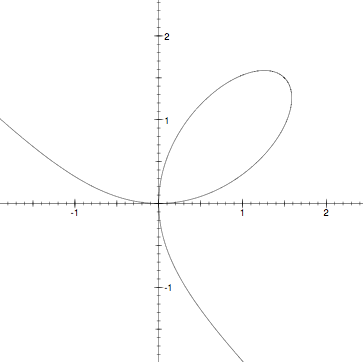Your plotter will attempt to find the points in a region that satisfy a given equation. The trick is to interpret the equation as a function of x and y which has zeros at the places that the equation is true. For example, the equation above is true wherever the function f(x,y) = x3 + y3 - 3xy is equal to zero. Fortunately, you just implemented interval arithmetic in PS3, which is useful for efficiently finding function zeros. Of course, you'll want to be sure to fix any bugs that you had in PS3, because otherwise there will be bugs in PS4.

The strategy for finding zeros is as in PS3: evaluate the function on a region of its domain (the plane) using interval arithmetic. If the result interval contains zero, there may be a zero of the function within that region. We then recursively subdivide this region into smaller subregions, and search for a zero within them. There is more than one way to subdivide into subregions, but the rule of thumb is that the subregions should be similar in size. If the interval doesn't contain zero, the function cannot either, because interval arithmetic is conservative.

For example, consider the equation y = x - 1, plotted on the rectangle from (-8, -8) to (8, 8). We want to plot the zeros of the function y - x + 1. Evaluating this function on the intervals x = [-8,8] and y = [-8,8], we get:

[-8, 8] - [-8, 8] + [1, 1] = [-15, 17].

The resulting interval [-15, 17] contains zero. Thus, we subdivide the x and y regions and recurse.

### Generating lines:

Your program will continue to refine the subregions containing solutions to the function until it reaches a predefined maximum recursion depth. At maximum depth, the program still has a small box that presumably contains a zero. The program then draws a line to approximate the location of the zero. This is done by checking for zeros along the four sides of the box, with a similar recursive approach. Once zeros are located to within the required numerical precision, lines are drawn connecting the edge zeros.

### Implementation

We have provided you some framework for this project. It's up to you to implement two modules: `Lines` and `Plotter`.

Lines will implement at least a signature with at least one operation:

```signature LINES = sig

type lines
type interval = Interval.interval

(* findLines(e, i1, i2, depth) generates lines representing the
* a plot of the equation e over the x,y ranges defined by the
* intervals i1 and i2. The maximum recursion depth is depth. *)
val findLines: Eqn.expr * interval * interval * int -> lines
end
```

Plotter will implement the signature:

```signature PLOTTER = sig

(* plot(lns, stream) writes a series of PostScript commands
* to stream, where each command draws a line represented by lns. *)
val plot: Lines.lines * TextIO.outstream -> unit
end
```

You will use `Lines.findLines` to generate a representation of all the lines to be plotted. How you choose to represent lines is up to you. You may want to add more operations to the `LINES` signature; think carefully before doing so.

### PostScript output

The output of the plotter program is a program written in the PostScript programming language, written to the file out.ps. PostScript is a stack-based programming language that is understood by many printers and can be converted to other formats such as PDF. To help you generate PostScript, we have provided PostScript code that draws the axes for the plot and that can draw lines between two points on the plot. Your job is to generate PostScript code that can be inserted into the template code found in the skel.ps.

Three functions are defined in skel.ps that your code can use. (Note that as a stack-based language, PostScript applies functions in postfix order: the function name comes after its argument.)

• `x0 x1 y0 y1 setbounds`

Set up the plot to have the x range [x0,x1] and the y range [y0,y1], and draw the axes and labels for the plot.

• `x0 y0 x1 y1 drawline`

Draw a line on the plot between (x0,y0) and (x1,y1) Requires that the plot is already set up.

• `x0 y0 x1 y1 filledbox`

Draw a solid black box in the plot, filling all points in [x0,x1]×[y0,y1]. Requires that the plot is already set up. This function may be useful in developing and debugging your code.

For example, the following code will generate a plot with a single line on it:
```0 1 0 1 setbounds
0 0 1 1 drawline
```

At the end of the PostScript code, the command `showpage` tells the printer that the page is complete. The provided main program already generates this for you.

You will implement `Plotter.plot`, which generates the PostScript code to be inserted into the template. If you want to explore or modify skel.ps, you can, but this is not necessary. For more information regarding PostScript check out http://en.wikipedia.org/wiki/PostScript.

You will call your plotting code from the `main` function in relplot.sml

Compile your code using `sml sources.cm`. This will generate a binary `relplot.x86-<OS>`, which executes the `main` function in relplot.sml. To run this program, type:

sml @SMLload=relplot.x86-<OS> <expr> <xMin> <yMin> <xMax> <yMax> [<depth>]

If you are running on a non-x86, you'll need to give a different extension for the file to load. You can write a script (.bat) file to do all this for you if you like.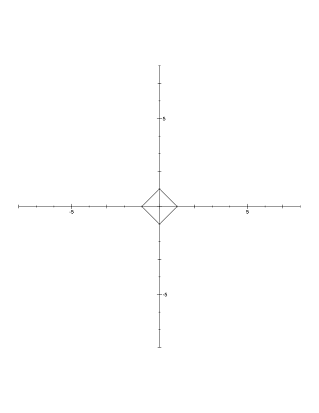X^2 + Y^2 = 1 depth = 1X^2 + Y^2 = 1 depth = 2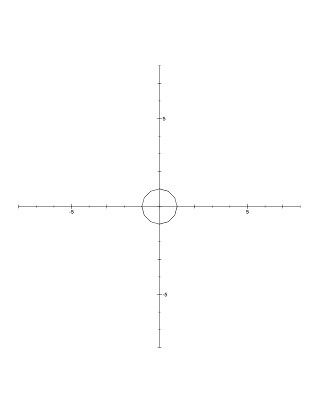X^2 + Y^2 = 1 depth = 5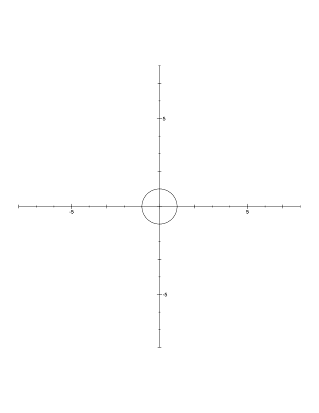X^2 + Y^2 = 1 depth = 7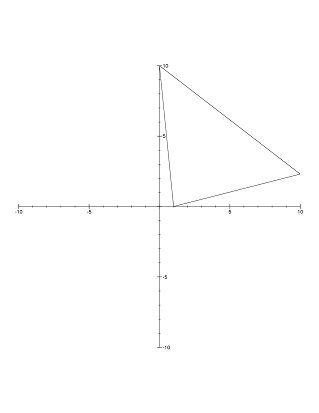Y = |ln X| depth = 1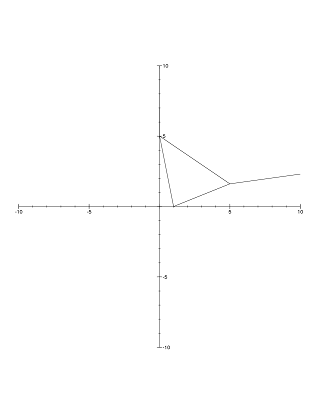Y = |ln X| depth = 2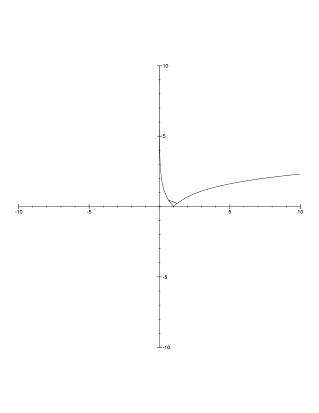Y = |ln X| depth = 5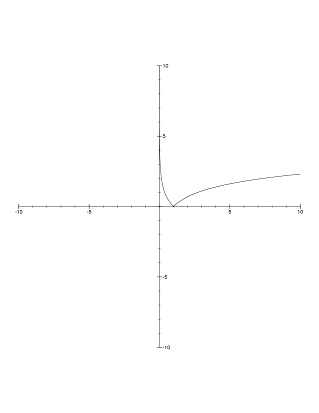Y = |ln X| depth = 8

Here are some more equations you may find interesting to plot and that you can test your code on:

A “flower”: `(sqrt(x^2 + y^2) - 1/2)^5 = 4yx^3 - 4xy^3`
A “Jellyfish”: `((xy(x-y)(x+y)(x^2+y^2-4))^2 - 1) = 1`

CVS: We ask you to use CVS while developing code with your partner. CVS is a popular, convenient versioning system that allows you and your partner to share code in a common repository. It allows you to share common code, commit your code into the repository, or update your version with the changes of your partner.

To do: Submit a file log file cvslog showing your cvs activity during the assignment. For this, look into the "cvs log" command. Submitting photos of you and your partner exchanging a flash drive does not count.

## Part 2: Proving Correctness

Prove that the following code finds the minimum value in a tree t, or `NONE` in the case of an empty tree. Hint: Prove that the specification of least' is correct.

```datatype tree = Node of (tree * int * tree) | Null

fun least(t:tree): int option =
let
(* least'(t, theLeast) is NONE if t is Null and theLeast is NONE.
* Otherwise, if theLeast is SOME(x), least'(t, theLeast) is SOME(y),
* where y is the minimum over all elements in t and x *)
fun least'(t: tree, theLeast: int option) =
case (t, theLeast) of
(Null, _) => theLeast
| (Node(l,v,r), NONE) => least'(l, least'(r,SOME(v)))
| (Node(l,v,r), SOME(lst)) =>
least'(l, SOME(Int.min(lst, valOf(least'(r,SOME(v))))))
in
least'(t, NONE)
end
```

To do: Submit part2.txt

## Part 3: Running Time 1

Consider the following recurrence defining T(n):
 For n > 1, T(n) = H(n) + T(n/2) H(n) = H(n−1) + 1 T(1) = H(1) = 1
1. Find the closed-form solution for T(n), where n is a power of 2.
2. Prove it correct by induction.

## Part 4: Running Time 2

Consider the function `fast`:
```fun fast(lst: int list): int =
case lst of
[] => 0
| [x] => x
| _ => let val (l1: int list, l2: int list, _) =
foldl
(fn (x:int, (l1:int list, l2:int list, toggle: bool)) =>
if toggle then (l1@[x], l2, not toggle)
else (l1, l2@[x], not toggle))
([], [], false)
lst
in
(fast l1) + (fast l2) + (fast l1) + (fast l2)
end
```
1. Write a recurrence for the run time T(n) of the function `fast`.
2. Prove that the solution to this recurrence is O(n2 lg n) where n is the length of `lst`.

To do: Submit part4.txt